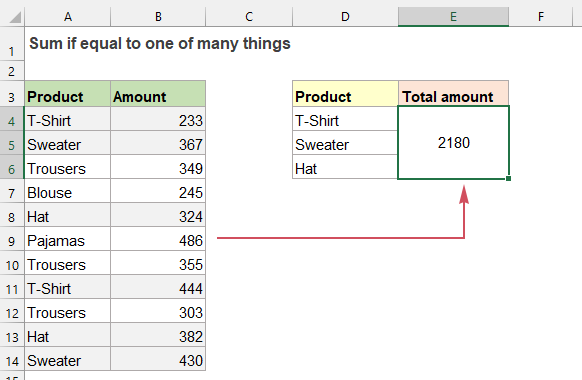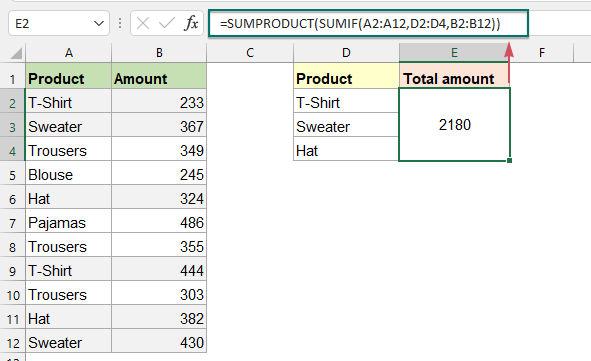## Sum if equal to one of many things in Excel

It may be easy for us to sum values based on a given criteria by using the SUMIF function. But, sometimes, you may need to sum values based on a list of items. For example, I have a data range which products are listed in Column A, and the corresponding sale amounts are listed in Column B. Now, I want to get the total amount based on the listed products in range D4:D6 as below screenshot shown. How to solve this problem quickly and easily in Excel?#### Sum if equal to one of many things with SUMPRODUCT and SUMIF functions

If you need to sum values when their corresponding cells are equal to one of many things, you can create a formula based on the SUMPRODUCT and SUMIF functions, the generic syntax is:

=SUMPRODUCT(SUMIF(range,criteria_range,sum_range))
• range: The range of cells to match with the criteria range;
• criteria_range: The range contains the values you are using as criteria;
• sum_range: The range of values where sum is required.

To solve the above problem, please apply the below formula, and then press Enter key to get the result:

=SUMPRODUCT(SUMIF(A2:A12,D2:D4,B2:B12))##### Explanation of the formula:

=SUMPRODUCT(SUMIF(A2:A12,D2:D4,B2:B12))

• SUMIF(A2:A12,D2:D4,B2:B12): SUMIF function returns three sums for each items in criteria_range (D2:D4) of their corresponding amounts in B2:B12. So, you will get the result as this: {677;797;706}.
• SUMPRODUCT(SUMIF(A2:A12,D2:D4,B2:B12))=SUMPRODUCT({677;797;706}): The SUMPRODUCT function sums all items in the array and returns a final result: 2180.

#### Relative function used:

• SUMPRODUCT:
• The SUMPRODUCT function can be used to multiply two or more columns or arrays together, and then get the sum of products.
• SUMIF:
• The SUMIF function can help to sum cells based on one criterion.

#### More articles:

• Sum If Cells Contain Specific Text In Another Column
• This tutorial will show you how to sum values if cells contain specific or partial text in another column. Take the below data range as an example, to get the total amounts of the products containing the text “T-Shirt”, both the SUMIF function and SUMPRODUCT function can deal with this task in Excel.
• Sum Smallest Or Bottom N Values In Excel
• In Excel, it is easy for us to sum a range of cells by using the SUM function. Sometimes, you may need to sum the smallest or bottom 3, 5 or n numbers in a data range as below screenshot shown. In this case, the SUMPRODUCT together with the SMALL function can help you to solve this problem in Excel.

### The Best Office Productivity Tools

#### Kutools for Excel - Helps You To Stand Out From Crowd

 Popular Features: Find, Highlight or Identify Duplicates  |  Delete Blank Rows  |  Combine Columns or Cells without Losing Data  |  Round without Formula ... Super VLookup: Multiple Criteria  |  Multiple Value  |  Across Multi-Sheets  |  Fuzzy Lookup... Adv. Drop-down List: Easy Drop Down List  |  Dependent Drop Down List  |  Multi-select Drop Down List... Column Manager: Add a Specific Number of Columns  |  Move Columns  |  Toggle Visibility Status of Hidden Columns  |  Compare Columns to Select Same & Different Cells ... Featured Features: Grid Focus  |  Design View  |  Big Formula Bar  |  Workbook & Sheet Manager | Resource Library (Auto Text)  |  Date Picker  |  Combine Worksheets  |  Encrypt/Decrypt Cells  |  Send Emails by List  |  Super Filter  |  Special Filter (filter bold/italic/strikethrough...) ... Top 15 Toolsets:  12 Text Tools (Add Text, Remove Characters ...)  |  50+ Chart Types (Gantt Chart ...)  |  40+ Practical Formulas (Calculate age based on birthday ...)  |  19 Insertion Tools (Insert QR Code, Insert Picture from Path ...)  |  12 Conversion Tools (Numbers to Words, Currency Conversion ...)  |  7 Merge & Split Tools (Advanced Combine Rows, Split Excel Cells ...)  |  ... and more

Kutools for Excel Boasts Over 300 Features, Ensuring That What You Need is Just A Click Away...#### Office Tab - Enable Tabbed Reading and Editing in Microsoft Office (include Excel)

• One second to switch between dozens of open documents!
• Reduce hundreds of mouse clicks for you every day, say goodbye to mouse hand.
• Increases your productivity by 50% when viewing and editing multiple documents.
• Brings Efficient Tabs to Office (include Excel), Just Like Chrome, Edge and Firefox.